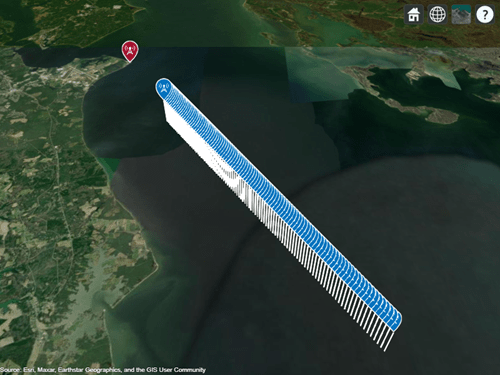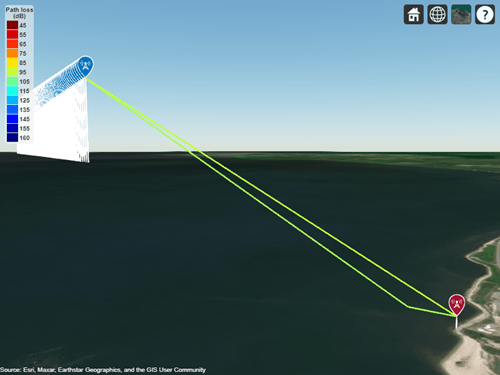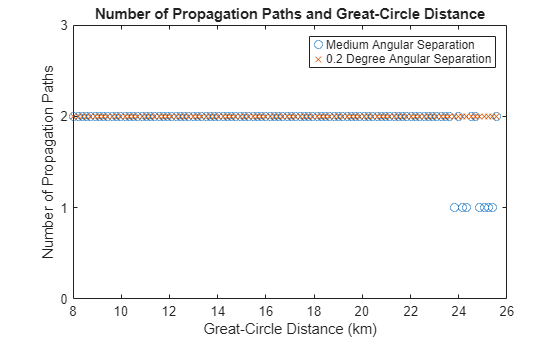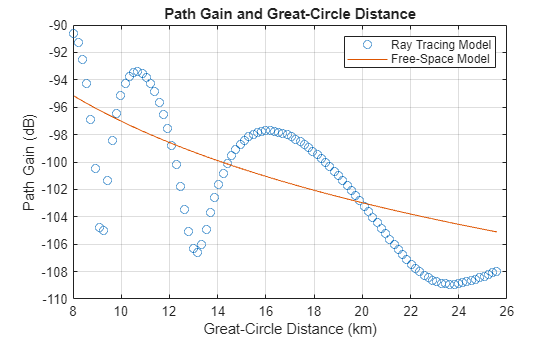# Analyze Communication Link for Aircraft over Seawater Using Ray Tracing

Use ray tracing to predict the path gain and signal strength of a radio link for an aircraft flying over seawater. Then, compare the analysis with the measured results reported in Multipath Interference for In-Flight Antenna Measurements .

### Define Transmitter and Receiver Sites

Create a transmitter site above the Chesapeake Bay, off the coast of Maryland, that uses a vertical half-wavelength dipole antenna. Specify the antenna height and the operational frequency using the values described by Figure 4 in . Ray tracing functions in MATLAB® require length units of meters, so convert the antenna height in Figure 4 from feet to meters.

```f = 167.5e6; txHeight = 62*0.3048; % ft -> m txAntenna = design(dipoleCylindrical,f); tx = txsite(Latitude=38.298138, ... Longitude=-76.374391, ... TransmitterFrequency=f, ... AntennaHeight=txHeight, ... Antenna=txAntenna);```

Specify latitude and longitude coordinates that represent the flight path of an aircraft. For this example, load variables from a MAT file that specify the coordinates of 100 points. The coordinates in the file were generated using the `track2` (Mapping Toolbox) function.

`load("rxCoordinates.mat","rxLat","rxLon")`

Use the coordinates to create 100 receiver sites that use vertical half-wavelength dipole antennas. Specify the antenna heights and operational frequencies based on the same figure in .

```rxHeight = 5000*0.3048; % ft -> m rxAntenna = design(dipoleCylindrical,f); rx = rxsite(Latitude=rxLat, ... Longitude=rxLon, ... Antenna=rxAntenna, ... AntennaHeight=rxHeight);```

Visualize the transmitter and receiver sites using the Site Viewer. Model the conditions in  by removing the terrain data from the Site Viewer.

```siteviewer(Terrain="none"); show(tx,ShowAntennaHeight=true) show(rx,ShowAntennaHeight=true)```### Plot Propagation Paths Using Ray Tracing

Create a ray tracing propagation model, which MATLAB represents using a `RayTracing` object. By default, the model uses the shooting and bouncing rays (SBR) method. Set the maximum number of path reflections to `1` and specify custom terrain materials using the seawater values reported in .

```pm = propagationModel("raytracing", ... MaxNumReflections=1, ... TerrainMaterial="custom", ... TerrainMaterialPermittivity=81, ... TerrainMaterialConductivity=4.64);```

Perform ray tracing analysis between the transmitter site and each receiver site. Then, plot only the propagation paths between the transmitter site and the first receiver site.

```rays = raytrace(tx,rx,pm); plot(rays{1})```

The ray tracing analysis finds a line-of-sight (LOS) path and a reflected path. Find a view that shows both paths by panning and zooming within the Site Viewer.By default, the ray tracing model uses an angular separation of "`medium`" which corresponds to an angular separation of approximately 0.54 degrees. For some scenarios, this angular separation might not find all viable propagation paths. Set the angular separation of the ray tracing model to 0.2 degrees and repeat the analysis.

```pm.AngularSeparation = 0.2; raysLessSeparation = raytrace(tx,rx,pm);```

Calculate the great-circle distances along the surface between the transmitter site and each receiver site.

`range = distance(tx,rx,"greatcircle");`

For each angular separation, plot the number of propagation paths against the great-circle distances. Medium angular separation finds two paths for most distances. By contrast, 0.2 degree angular separation finds two paths for all distances.

```numRays = cell2mat(cellfun(@numel,rays,"UniformOutput",false)); numRaysLessSeparation = cell2mat(cellfun(@numel,raysLessSeparation,"UniformOutput",false)); figure plot(range/1e3,numRays,"o",DisplayName="Medium Angular Separation") hold on plot(range/1e3,numRaysLessSeparation,"x",DisplayName="0.2 Degree Angular Separation") hold off ylim([0 3]) yticks([0 1 2 3]) title("Number of Propagation Paths and Great-Circle Distance") xlabel("Great-Circle Distance (km)") ylabel("Number of Propagation Paths") legend```### Predict Path Loss

Predict the path loss at each receiver site by calculating the phasor sums of the LOS and reflected paths.

```rayPL = nan(size(rx)); for i = 1:numel(rx) E = sum(exp(1i*[raysLessSeparation{i}.PhaseShift])./10.^([raysLessSeparation{i}.PathLoss]/20)); rayPL(i) = -20*log10(abs(E)); end```

Calculate the free-space path loss at each receiver site.

```distanceFSPL = sqrt(range.^2 + (txHeight-rxHeight).^2); FSPL = fspl(distanceFSPL,physconst("LightSpeed")/f);```

Plot the predicted ray tracing path gains (the negatives of the path losses) and the free-space path gains against the great-circle distances. The ray tracing model shows constructive and destructive interference between the LOS and reflected paths. By contrast, the free-space model shows only the LOS path. The ray tracing results closely match the measured and calculated data in Figure 4 of  after converting path gains to height gains. To predict path losses for distances significantly longer than the distances shown in the figure, the model also needs to account for atmospheric refraction.

```figure plot(range/1e3,-rayPL,"o",DisplayName="Ray Tracing Model") hold on plot(range/1e3,-FSPL,DisplayName="Free-Space Model") hold off grid on title("Path Gain and Great-Circle Distance") xlabel("Great-Circle Distance (km)") ylabel("Path Gain (dB)") legend```### Predict Signal Strength

Predict the received signal strength power in decibels referred to 1 milliwatt (dBm) for the ray tracing and free-space models. Assume a transmit power of `10` watts. When you specify a ray tracing propagation model as input, the `sigstrength` function incorporates multipath interference by using a phasor sum.

```tx.TransmitterPower = 10; ssRay = sigstrength(rx,tx,pm); ssFS = sigstrength(rx,tx,"freespace");```

Plot the predicted signal strength power and the free-space signal strength power against the great-circle distances. The ray tracing results shows constructive and destructive interference between the LOS and reflected paths. By contrast, the free-space results show only the LOS path. To predict signal strength power for distances significantly longer than the distances shown in the figure, the model also needs to account for atmospheric refraction.

```figure plot(range/1e3,ssRay,"o",DisplayName="Ray Tracing Model") hold on plot(range/1e3,ssFS,DisplayName="Free-Space Model") hold off grid on title("Received Power and Great-Circle Distance") xlabel("Great-Circle Distance (km)") ylabel("Received Power (dBm)") legend```### References

 Balanis, C., R. Hartenstein, and D. DeCarlo. “Multipath Interference for In-Flight Antenna Measurements.” IEEE Transactions on Antennas and Propagation 32, no. 1 (January 1984): 100–104. https://doi.org/10.1109/TAP.1984.1143183.